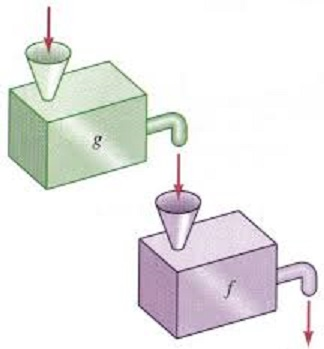# Section 3.5 - Algebraic Operations on Functions

Section Objectives

1. Compute sums, differences, products, and quotients of functions.
2. Compute a composition of functions.
3. Write a function as a composition of functions.
4. Solve problems involving operations on functions.

### Combining functions

Besides transforming individual functions, two or more functions can be combined in lots of ways to produce new functions.

The most basic combinations of functions come from the four basic arithmetic operations.

• $(f+g)(x)=f(x)+g(x)$
• $(f-g)(x)= f(x)-g(x)$
• $(fg)(x)=f(x) \cdot g(x)$
• $\displaystyle \left( \frac{f}{g} \right) (x) = \frac{f(x)}{g(x)}$, provided that $g(x) \ne 0$

#### Examples

• Given the following table of values, compute $(f+g)(2)$, $(f-g)(3)$, and $(fg)(1)$.

• Let $f(x)=x^2-7x+1$ and $g(x) = x^3$. Compute $(f/g)(2)$. What about $(f/g)(0)$?

• Sketch the graphs of $f(x)=x-3$ and $g(x)=x^2+2$. Use the graphs to evaluate $(f+g)(1)$. What about $(f-g)(0)$?

### Compositions

Another useful and important way of combining functions is to form the composition. Functions are composed when the output of one function is used as the input for a second function.

The composition of $f$ and $g$, written $f \circ g$, is the new function $(f \circ g)(x) = f(g(x))$.#### Examples

• Let $f(x)=x^2-5x$ and let $g(x)=x^3$. What function is $f \circ g$? What about $g \circ f$? What about $f \circ f$?

• Refer to the table above. Compute $(f \circ g)(0)$.

• Let $h(x) = (x^2+1)^5$. Find two functions $f$ and $g$ so that $h(x) = (f \circ g)(x)$.

• Let $g(x)=\sqrt{5x+25}$ and $h(x)=x-4$. Find $g \circ h$ and determine its domain.

• Let $\displaystyle f(x)=\frac{2}{x+3}$ and $\displaystyle g(x)=\frac{1}{x-2}$. Find and completely simplify $f \circ g$. What is the domain of the composition?

• A large spherical balloon is being inflated so that its radius, in feet, after $t$ minutes is given by $r(t)=2t+3$. Find its volume as function of $t$.

• An object is cooling in such a way that its temperature in degrees Celsius after $t$ minutes is given by $C=80-0.01t^2$. The formula $\displaystyle f= \frac{9}{5}c+32$ is used to convert from temperatures in Celsius to Fahrenheit. Find the temperature of the object in degrees Fahrenheit at time $t$.